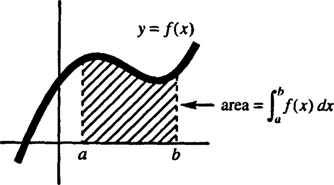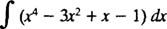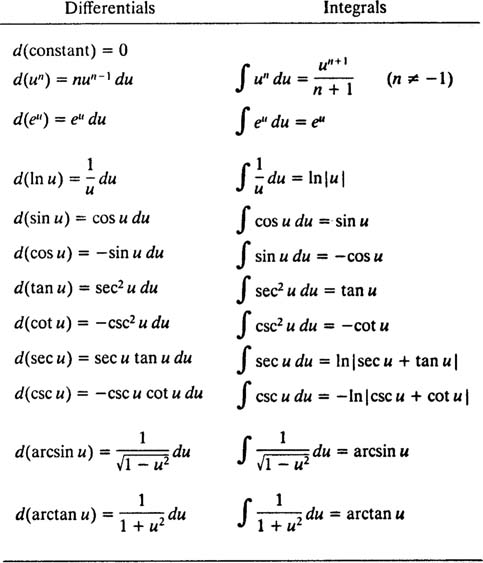## Integration

Indefinite integration means antidifferentiation; that is, given a function ƒ( x), determine the most general function F( x) whose derivative is ƒ ( x). The symbol for this operation is the integral sign, ∫, followed by the integrand (the function to be integrated) and differential, such as dx, which specifies the variable of integration.

On the other hand, the fundamental geometric interpretation of Definite integration is to compute an area. That is, given a function ƒ( x) and an interval axb in its domain, the definite integral of ƒ from a to b gives the area bounded by the curvey y = ƒ ( x), the x axis, and the vertical lines x = a and x = b. The symbol for this operation is the integral sign with limits of integration ( a and b), ƒ a b , followed by the function and the differential which specifies the variable of integration.Figure 1

From their definitions, you can see that the processes of indefinite integration and definite integration are really very different. The indefinite integral of a function is the collection of functions which are its antiderivatives, whereas the definite integral of a function requires two limits of integration and gives a numerical result equal to an area in the xy plane. However, the fact that both operations are called “integration” and are denoted by such similar symbols suggests that there is a link between them.

The Fundamental Theorem of Calculus says that differentiation (finding the slope of a curve) is the inverse operation of definite integration (finding the area under a curve). More explicitly, Part I of the Fundamental Theorem says that if a function is integrated (to form a definite integral with a variable upper limit of integration), and the result is then differentiated, the original function is recovered; that is, differentiation “undoes” integration. Part II gives the connection between definite and indefinite integrals. It says that a definite integral can be computed by first determining an indefinite integral (so computing the area under a curve is done by antidifferentiating).

• The Fundamental Theorem of Calculus (Part I):
• Ifi> ƒ is continuous, then d dxx a ƒ( t) dt = ƒ( x).
• The Fundamental Theorem of Calculus (Part II):
• If ƒ is continuous with antiderivative F, then ∫ b a ƒ( x) dx = F( b) − F( a).

Example 1: Evaluate the integralUsing the first integration formula in Table 1, every function whose derivative equals ƒ( x) = x 4 − 3 x 2 + x − 1 is given bywhere c is an arbitrary constant.

TABLE 1 Differentiation and Integration Formulas